# Testing Benford’s Law using Python

In Episode 4, Season 1 of the Netflix series Connected, Latif Nasser explored Benford’s law.  In the episode, Benford’s law is described as “a law of numerical probability that applies to classic music, contemporary social media, tax fraud and perhaps the entire universe”. I was hooked and tried to figure out if there would be a relatively straightforward way to better understand why this law is so pervasive, just using Python. As it turns out, there is. If you are interested to know more, follow me in this little computational adventure 🙂

You will need some basic imports before we can begin

import math
import random
import pandas as pd
import matplotlib.pyplot as plt

## Step 1: What is Benford’s law?

Basically, Benford’s law states that the probability that the first digit of any number is a specific figure is given by

$$P(digit) = log_{10}(digit+1) – log_{10} (digit) = log_{10} \left( \frac{digit+1}{digit} \right)$$

So, according to Benford’s law, the likelihood that any number you are currently looking at starts with a “1” is:

$$P(digit=1) = log_{10} \left( \frac{1+1}{1} \right) = log_{10}(2) = 0.301 = 30.1\%$$

Similarly, the probability that the same number actually starts with a “2” would be

$$P(digit=2) = log_{10} \left( \frac{2+1}{2} \right) = log_{10}(\frac{3}{2}) = 0.176 = 17.6\%$$

You can continue like this until digit 9.

Here is a little function that will calculate this likelihood for you:

def benford_proportion(first_digit):
"""
returns the likelihood (between 0 and 1) that the first digit
of any number you are looking at starts with n, according to
Benford's law. n should be between 1 and 9
"""
if not isinstance(first_digit, int) or first_digit < 1 or first_digit > 9:
raise ValueError("n should be an integer between 1 and 9")

likelihood = math.log10((first_digit+1)/first_digit)
return likelihood


Now let’s plot these probabilities for digits 1 to 9 using Python

def plot_benford_proportions():

fig, axs = plt.subplots(figsize=(12,4))

xv = range(1, 10)
yv = [benford_proportion(first_digit) for first_digit in xv]

axs.bar(xv, yv, color='#0A75AD', label="Proportion according to Benford's law")

plt.xlabel("first_digit", fontsize=14)
plt.ylabel("Likelihood of being first digit", fontsize=14)
plt.legend(fontsize=14)
plt.xticks(ticks=xv, labels=xv)
plt.savefig("img/01_benford_proportions.png", dpi=200)
plt.show()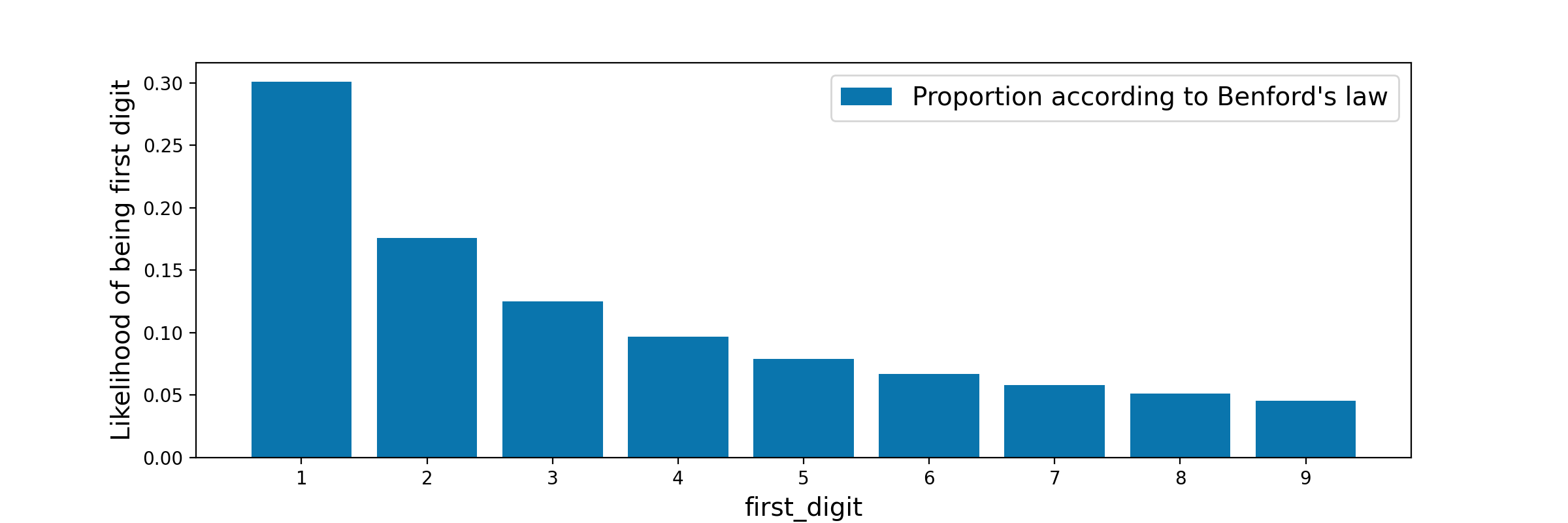So as you can see, a “1” as a first digit would be much more likely than a “2”, which would be more likely than a “3”, etc.

## Step 2: Is Benford’s law true for random numbers?

We’ll let’s try and find out! To make it easier to plot, let’s create a function that will return a dataframe filled with random numbers. Let’s also group them by first digit and count them.

def generate_data(vmin=1, vmax=999999, total_numbers=500):
""" Generate random integers between vmin and vmax of length total_numbers.
Group them in a dataframe and count the number of occurences of their first digit.
Also compute the proportions of their first digit.
"""
df = pd.DataFrame(columns=['first_digit', 'count'])

for i in range(total_numbers):
#generate a random integer between vmin and vmax
x = random.randint(vmin, vmax)
# extract the first digit
d = int(str(x))
# create a row for this digit in the dataframe
df.loc[i] = [d, 1]

# group the daframe by first digit, count the number of occurences of each first digit
df = df.groupby('first_digit').sum().reset_index()
df = df.sort_values(by='first_digit')

# also compute the proportions of each digit
df['true_proportion'] = df['count']/df['count'].sum()
return df


Here is the result with 10 random numbers generated between 1 and 999999, and grouped by first digit. As you can see, we got two random numbers starting with a ‘5’  (so 20% of the total of 10 random numbers generated), another two numbers starting with a ‘6’, and another two random number starting with a ‘7’.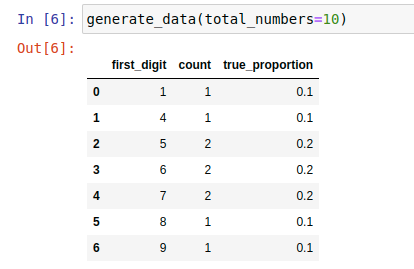Of course, one cannot really make sense of random events unless one sees A LOT of them, so let’s generate these counts for n=100, n=1,000, and n=10,000 and plot them against the expected Benford’s proportions.

def plot_digit_counts(df_list):

fig, axs = plt.subplots(nrows=len(df_list), ncols=1, figsize=(12, 10), sharex=True)

# Expected proportion of first digits if the dataset followed Benford's Law
xv = range(1, 10)
benford_prop = [benford_proportion(n) for n in xv]

# One subplot per set of random numbers generated
for i in range(len(df_list)):

# Length of the dataset
data = df_list[i]

# Plot the actual proportions versus the Benford proportions
axs[i].bar(data['first_digit'], data['true_proportion'], label='Actual Proportion')

axs[i].plot(
xv,
benford_prop,
'o-',
color='red',
label="Expected proportion by Benford's Law"
)

axs[i].set_title('{0} Random Numbers Generated'.format(data['count'].sum()))
axs[i].legend(loc='upper right')

plt.xticks(ticks=xv, labels=xv)
plt.show()

# Let's generate these numbers and plot them
plot_digit_counts([
generate_data(total_numbers=100),
generate_data(total_numbers=1000),
generate_data(total_numbers=10000)
])


Give it a few seconds to run and you should see a result similar to mine below: the more random numbers you generate, the more uniform the proportion of getting any digit between 1 and 9 as a  first digit.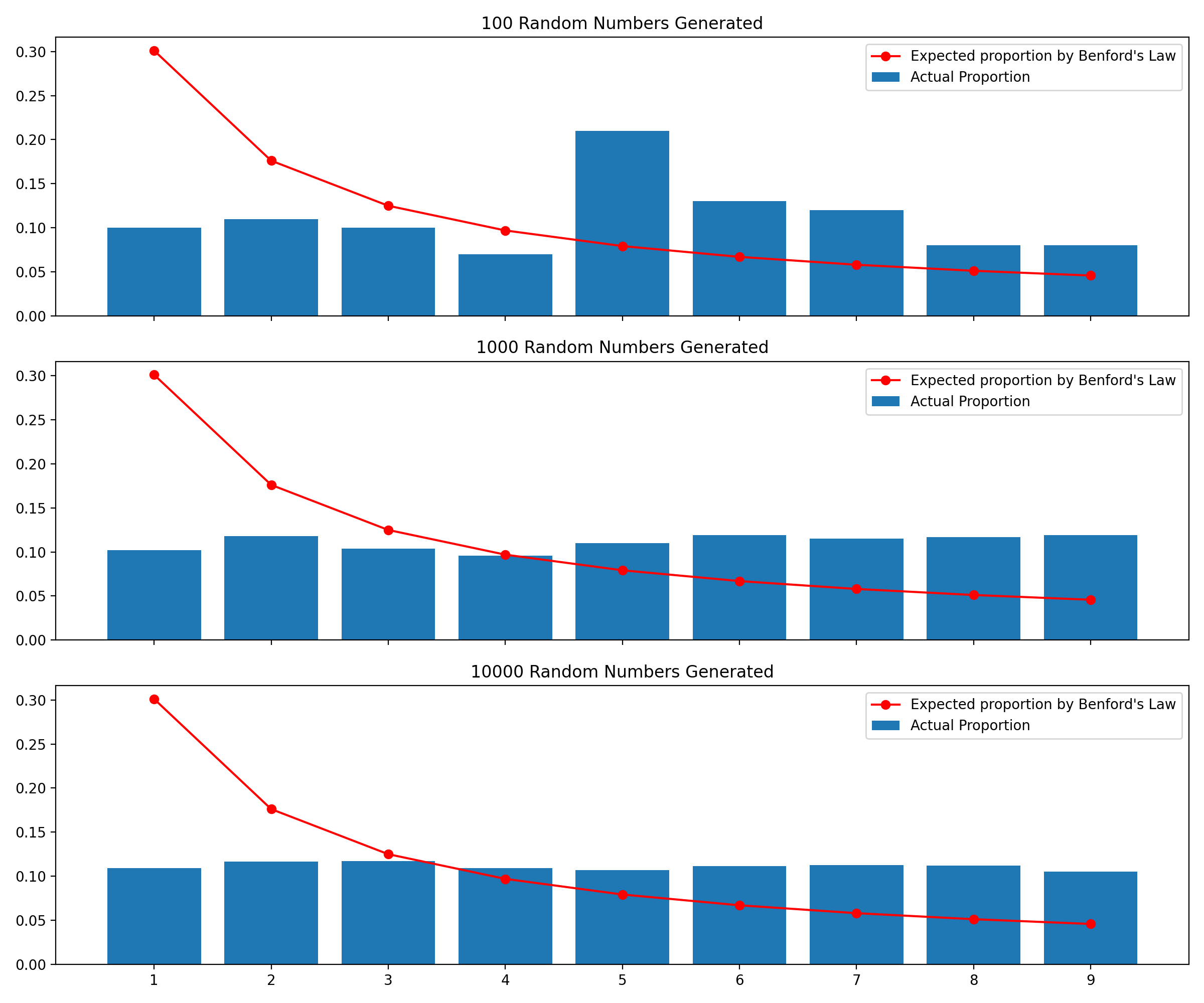So this seems disappointing at first sight: random numbers DO NOT follow Benford’s law. But then what does??

## Step 3: Benford’s Law with Exponential Growth Situations

 Remember the exponential function? So it is well known that the exponential is a pervasive function in our world as well. The exponential applies to anything that starts with 1, then becomes 2. From then 2 becomes 4, 4 becomes 8, etc. Think egg division. Also think bacterial growth.  Also think nuclear fission. Think about anything that compounds over time basically. Don’t believe me? Check this link out for just a few examples.  So the next thing I wanted to know was whether exponential processes follow Benford’s law. Here is a basic way to test this assumption: (1) Generate many distinct populations (2) For each population (2.1) Choose a maximum number of generations that you’ll let this population grow to (2.2) Use a basic population growth equation where the number of individuals at generation n_gen = 2generationSo we’ll get 2¹ = 2 people at generation 1, 2²=4 people at generation 2, etc (2.3) Store the first digit of the total number of individuals, since we want to know whether that first digit follows Benford’s law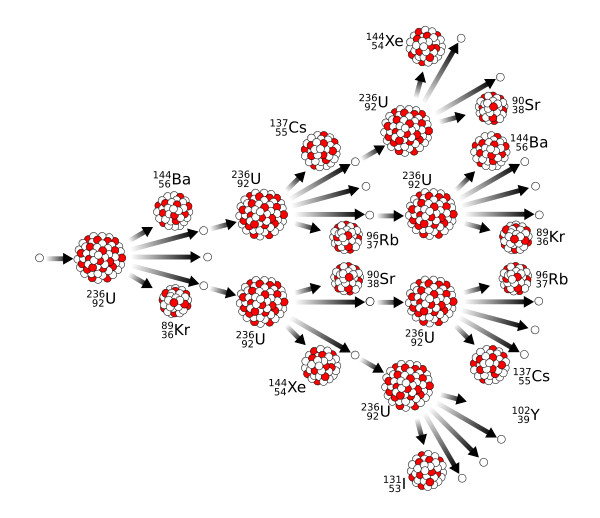Nuclear fission: follows the exponential law too

Here is a code snippet to do this:

def grow_population(n_generations):
"""
Unlimited population growth: at each generation, the number
of individuals is 2^generation
"""
return math.pow(2, n_generations)

def generate_simple_population_data(ngen_min=1, ngen_max=25, n=500):
"""
Generate simple population growth datasets. More specifically:
- generate n populations
- let each population grow for a random number of generations ngen
- compute the total number of individuals when the population reaches ngen
- get first digit of the total number of individuals
"""
# Final dataframe
df = pd.DataFrame(columns=['first_digit', 'count'])

# Generate n distinct populations
for i in range(n):
# choose the number of generations ngen at random
n_gen = random.randint(ngen_min, ngen_max)
# the total number of individuals when the population reaches ngen
population = grow_population(n_gen)
# get first digit of the total number of individuals
d = int(str(population))
# we are only interested in the first digit
df.loc[i] = [d, 1]

# group the daframe by first digit, count the number of occurences of each first digit
df = df.groupby('first_digit').sum().reset_index()
df = df.sort_values(by='first_digit')

# also compute the proportions of each digit
df['true_proportion'] = df['count']/df['count'].sum()
return df

Here are the results with different datasets of 100, 1,000 and 10,000 random populations following exponential growth

plot_digit_counts([
generate_simple_population_data(n=100),
generate_simple_population_data(n=1000),
generate_simple_population_data(n=10000)
])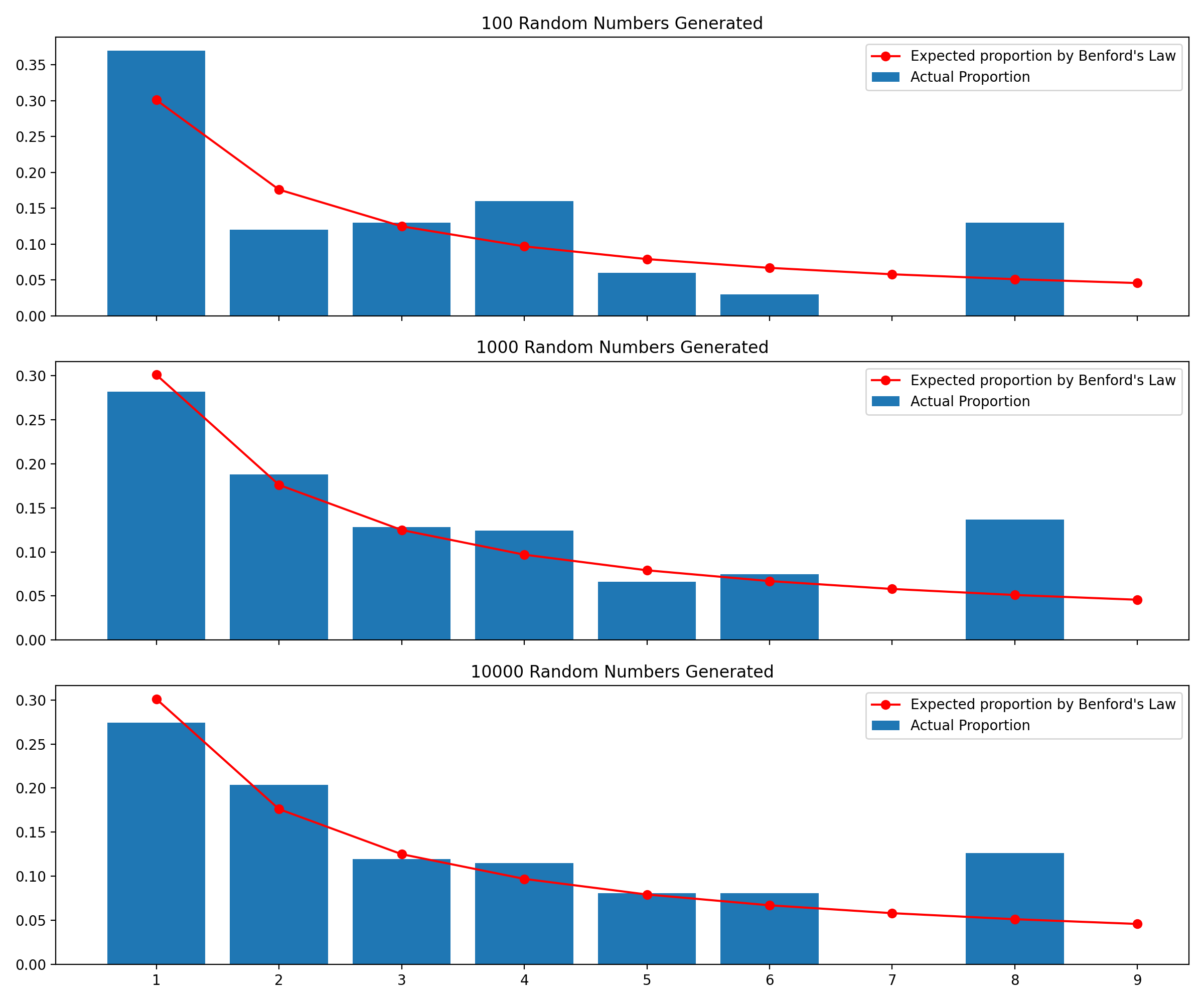So it starts a bit chaotic at first, but as the number of random populations generated increases, the actual proportions of first digits do start to follow Benford’s Law…except that there are no populations starting with digits ‘7’ and ‘9’. Why is that?

Well, remember that I’m generating my total population sizes using number of individuals = 2generation . It turns out that the first power of two that starts with a ‘7’ is 246 = 70,368,744,177,664, and the first power of two that starts with a ‘9’ is 253 = 9,007,199,254,740,992!

In my function generate_simple_population_data(), the maximum number of generations my little experiment generated was 25, so no wonder these leading digits never showed up.

But hey, this is encouraging! Benford’s Law is starting to show up in this idealized, extremely simple population growth model. But will it show up in a more realistic growth model?

## Step 4: Benford’s Law and the Malthusian growth model

 In 1798, the English economist Thomas Robert Malthus wrote his most influential book, “An Essay on the Principle of Population”. Essentially, Malthus said that it was not realistic to believe that population could increase exponentially forever, because more people = more mouths to feed, and food production does not increase exponentially every year. Malthus basically predicted that famine and poverty would increase in England unless birth rates were lowered. At that time, his book actually had a strong influence on many people, including Charles Darwin, who used it as inspiration to come up with his theory of Natural selection. Now what’s the link between Malthus and our current experiment? Well, as it turns out, Malthus came out with a growth model that’s a little bit more realistic than our simple 2generation function of Step 3. His model goes like this: $$P(t) = P_0 e^{rt}$$ Where: P(t) is the population at year t P0 is the initial population r is the population growth rate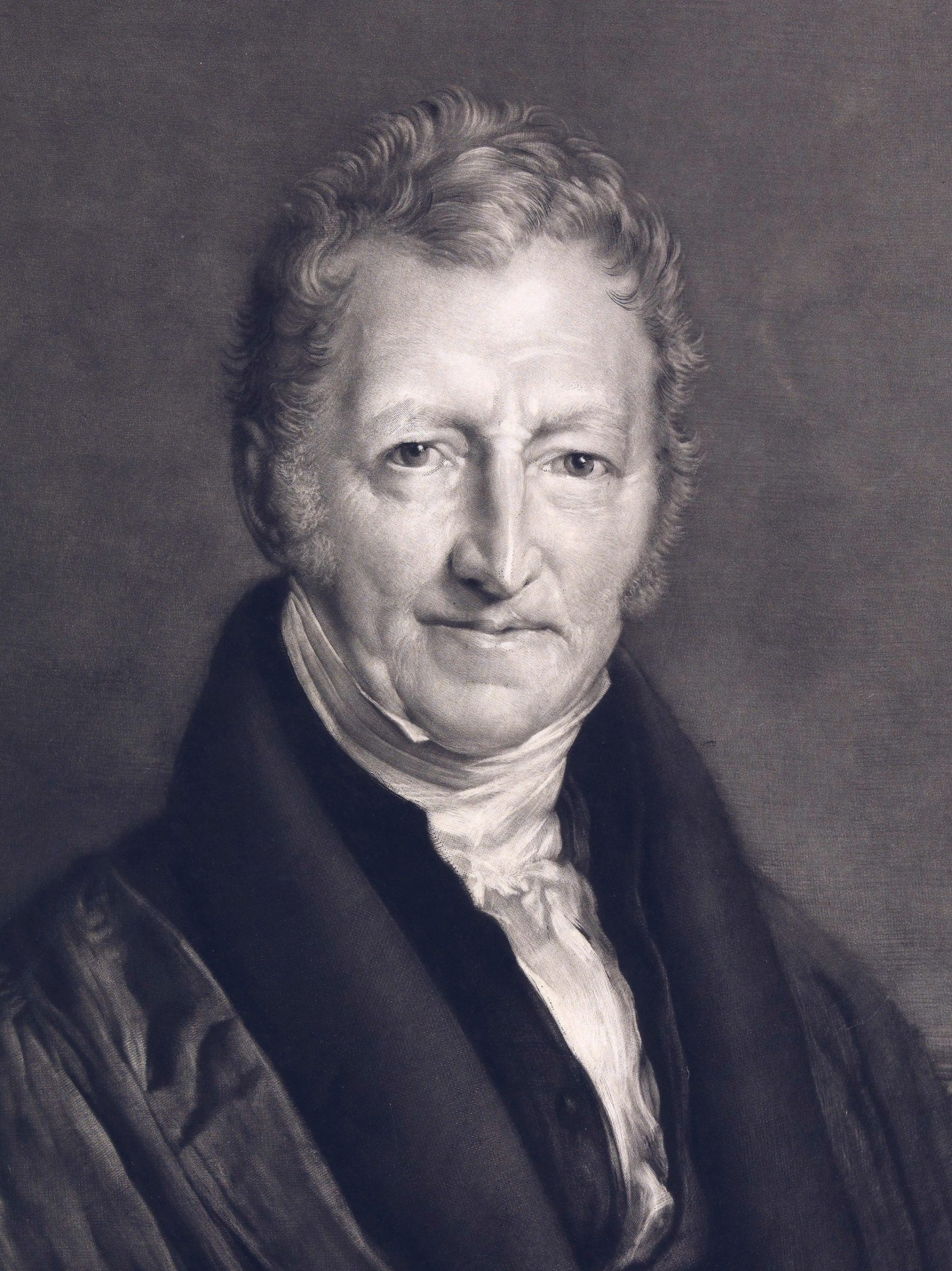Thomas Robert Malthus (Wikipedia page)

So let’s see how this can be simulated using Python. Similar as in Step 3, we will generate random independent populations. Each population will have a random time to grow (between 1 and 100 years).

For the growth rate r, this page from San Diego State University suggests r=0.349 to estimate the population of the U. S. from 1790 to 1860, and to make it even more variable, I will choose at random between 0.1 and 2.5. Feel free to test other values 🙂

def malthusian_growth(P0, r, t):
p = P0 * math.exp(r*t)
return p

def generate_malthusian_population_data(n=500):
"""
Generate n populations following the Mathusian growth model.
r is generated ar random using realistic ranges (between 0.5 and 2.5)
"""
df = pd.DataFrame(columns=['first_digit', 'count'])

for i in range(n):
# Generate P0 at random
P0 = random.randint(1, 1000000)
# Gererate r at random within realistic ranges 0.50 to 2.50
r = random.uniform(0.1, 2.5)
# Generate t at random between 1 and 100
t = random.randint(1, 100)

# Get malthusian population
p = malthusian_growth(P0, r, t)
d = int(str(p))

df.loc[i] = [d, 1]

# group the daframe by first digit, count the number of occurences of each first digit
df = df.groupby('first_digit').sum().reset_index()
df = df.sort_values(by='first_digit')

# also compute the proportions of each digit
df['true_proportion'] = df['count']/df['count'].sum()
return df

plot_digit_counts([
generate_malthusian_population_data(n=100),
generate_malthusian_population_data(n=1000),
generate_malthusian_population_data(n=10000)
])


And here is what happens when you create 100, 1000, then 10000 random populations using the Mathusian growth model. Behold Benford’s law in action!!Phew =) Took us a little time to get there, but it was worth it. I believe – from a quick Google search – that some research (and maybe even some proofs?) exist out there that link Benford’s Law and exponential growth, also to the exponential distribution as it seems (see here and here). But I didn’t look much further as this was just for fun. But if you have solid evidence to back this experiment, please send me a quick Linkedin message, would be glad to chat!